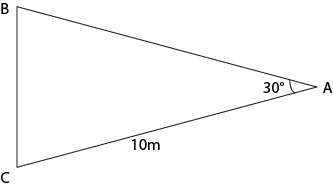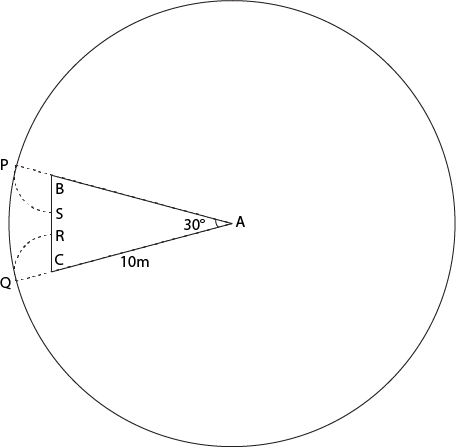### CAT 1998 Question Paper Question 55

Instructions

Answer the questions based on the following information. A cow is tethered at point A by a rope. Neither the rope nor the cow is allowed to enter ΔABC.
∠BAC = 30°
I(AB) = I(AC) = 10 mQuestion 55

# What is the area that can be grazed by the cow if the length of the rope is 12 m?

SolutionArea grazed by cow will be = Area of the larger circle - Sector APQ + Sector PBS + Sector CQR

Area of larger circle - Sector APQ = $$\pi r^2 - \frac{\pi r^2}{12}$$ = $$132 \pi$$

Sectors PBS and CQR are symmetrical and hence will have equal area.

In triangle ABC, AB = AC => ABC is isosceles triangle.

As A = 30° => B = C =75°

Hence, angle PBS = 180-75 = 105°

Hence, Area of sector PBS = 105/360 * $$\pi * 2^2$$ = $$7 \pi / 6$$

Thus area that can be grazed by the cow = $$132 \pi + 2*7 \pi /6$$ = $$403\pi /3$$